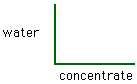Performance Stations in Math
Ratio and Proportion

by
Liliane Gauthier
Teacher / Educational Consultant

1. Ratio and Proportion

R-2, R-4, R6a

Materials:
or Rainbow cubes
grid paper

 1 Use the blocks to show 4 ratios that are equivalent to a ratio of 5 to 7. Record. You can use grid paper. 2 Give three examples of ratios in real-life situations. 3 Complete the activity sheet.

2. Ratio and Proportion

R-4, R-6b, A-10

Materials:
Can of frozen juice

1.What ratio of the water to concentrate is needed to make this juice?
2.Suppose you wanted to make more than one can. Fill in the chart below to show how much you need in each case.
concentrate1234567
juice
3.Use the graph paper to draw a graph in the first quadrant.4.You may choose to do this work using a spreadsheet on your computer.

3. Ratio and Proportion

R-11

Materials:
flyers and newspaper
calculator

 1 At one store hockey sock tape is sold at 6 rolls for \$10.86. At another store 8 rolls cost \$14.24. Which is the better buy? 2 Use the flyers and the newspapers to create 3 problems the involve unit pricing to calculate the best price. Solve the problems (show your work).

4. Ratio and Proportion

R-2, R-13 R-14, R-15

Materials:
10 x 10 grids
crayons

 1. Use the 10 x 10 grids to illustrate the following: a) a ratio of 1:5 b) 57% c) 0.37 d) 2/5 e) a ratio of 1:99 2. Use a 10 by 10 grid to design a logo in one color. Follow the lines in the grid. Diagonal lines are not allowed! Express the squares you colored as a a) percentage b) fraction c) decimal number d) ratio